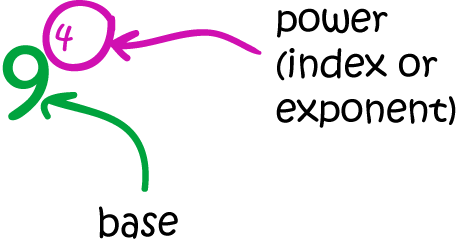# Definition of PowerThe power of a number tells us how many times we need to multiply it with itself to give the required value.

It is written as a little number above and to the right of the base number.

For example, $9^4 = 9 \times 9 \times 9 \times 9$. The power of $4$ tells us to multiply $9$ by itself $4$ times.

Other terms for "power" are "index" and "exponent".

### Description

The aim of this dictionary is to provide definitions to common mathematical terms. Students learn a new math skill every week at school, sometimes just before they start a new skill, if they want to look at what a specific term means, this is where this dictionary will become handy and a go-to guide for a student.

### Audience

Year 1 to Year 12 students

### Learning Objectives

Learn common math terms starting with letter P

Author: Subject Coach
You must be logged in as Student to ask a Question.